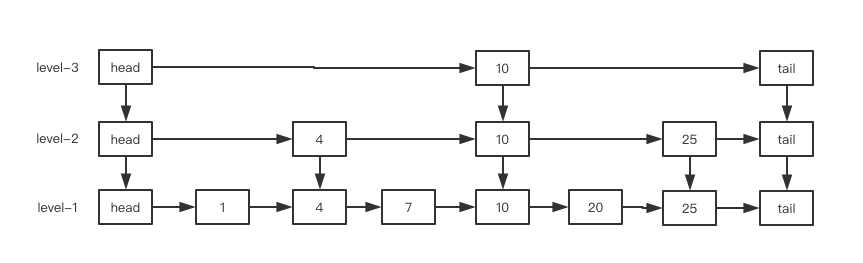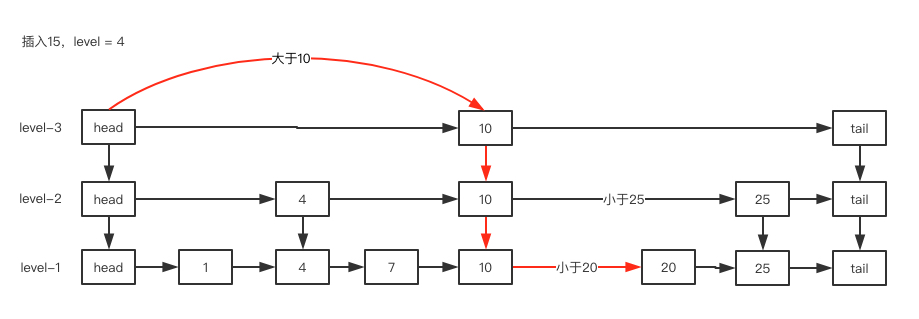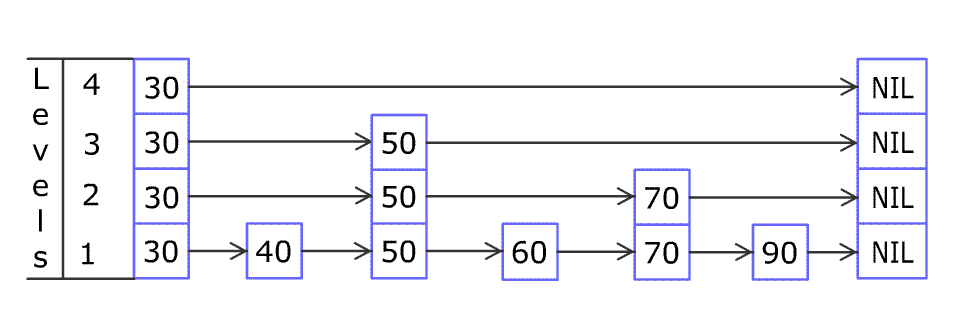## 引言

• 同步类容器都是线程安全的，比如Vector，HashTable，这些容器的同步功能其实是由Collections.Synchronized.**等工厂方法去创建实现的，底层机制无非是用传统的synchronized关键字给每个公共方法进行同步，使得容器再同一时间只有一个线程访问，这些古老的同步类容器已经有点不适应当今的高并发互联网需求
• 某些场景下可能要加锁来保护复合类操作，复合类操作，比如：迭代，跳转（按指定顺序查找当前元素的下一元素），以及条件运算，这些复合操作在多线程并发的修改容器时，可能会出现意外的行为，比如ConcurrentModificationExcepiton，当容器迭代的过程中，被其他线程并发的修改了内容，这是因为早期设计迭代器的时候，并没有考虑到并发修改的问题
• 单独的并发修改，比如remove还是线程安全的：

## 同步容器Vector和HashTable

``````//Vectorpublic synchronized int size() {};public synchronized E get(int index) {};//HashTable public synchronized V put(K key, V value) {};public synchronized V remove(Object key) {};
``````

``````public static void deleteVector(){    int index = vectors.size() - 1;    vectors.remove;}
``````

``````public static void deleteVector(){    synchronized  {        int index = vectors.size() - 1;        vectors.remove;    }}
``````

``````public static void foreachVector(){    synchronized  {        for (int i = 0; i < vectors.size {            System.out.println(vectors.get.toString;        }    }}
``````

## 并发容器CopyOnWrite

CopyOnWrite–写时复制容器是一种常用的并发容器，它通过多线程下读写分离来达到提高并发性能的目的，和前面我们讲解StampedLock时所用的解决方案类似：任何时候都可以进行读操作，写操作则需要加锁。不同的是，在CopyOnWrite中，对容器的修改操作加锁后，通过copy一个新的容器来进行修改，修改完毕后将容器替换为新的容器即可。

JDK中提供的并发容器包括CopyOnWriteArrayList和CopyOnWriteArraySet，下面通过CopyOnWriteArrayList的部分源码来理解这种思想：

``````//添加元素public boolean add {    //独占锁    final ReentrantLock lock = this.lock;    lock.lock();    try {        Object[] elements = getArray();        int len = elements.length;        //复制一个新的数组newElements        Object[] newElements = Arrays.copyOf(elements, len + 1);        newElements[len] = e;        //修改后指向新的数组        setArray(newElements);        return true;    } finally {        lock.unlock();    }}public E get(int index) {    //未加锁，直接获取    return get(getArray;}
``````

CopyOnWrite容器虽然在多线程下使用是安全的，相比较Vector也大大提高了读写的性能，但它也有自身的问题。

``````public class Tickets {

public static void main(String[] args) {
//初始化火车票池并添加火车票:避免线程同步可采用Vector替代ArrayList  HashTable替代HashMap

final Vector<String> tickets = new Vector<String>();
// 被Collections.synchronized**处理之后，map现在是线程安全的
//Map<String, String> map = Collections.synchronizedMap(new HashMap<String, String>());

for(int i = 1; i<= 1000; i++){
}

//      for (Iterator iterator = tickets.iterator(); iterator.hasNext();) {
//          String string = (String) iterator.next();
//          tickets.remove(20);
//      }
//单独的并发修改，比如remove还是线程安全的 ，remov并不是复合类操作
for(int i = 1; i <=10; i ++){
public void run(){
while(true){
if(tickets.isEmpty()) break;
}
}
}.start();
}
}
}
``````

## 并发容器ConcurrentHashMap

ConcurrentHashMap容器相较于CopyOnWrite容器在并发加锁粒度上有了更大一步的优化，它通过修改对单个hash桶元素加锁的达到了更细粒度的并发控制。在了解ConcurrentHashMap容器之前，推荐大家先阅读我之前对HashMap源码分析的文章–Java集合一
HashMap与HashSet，因为在底层数据结构上，ConcurrentHashMap和HashMap都使用了数组+链表+红黑树的方式，只是在HashMap的基础上添加了并发相关的一些控制，所以这里只对ConcurrentHashMap中并发相关代码做一些分析。``````final V putVal(K key, V value, boolean onlyIfAbsent) {    if (key == null || value == null) throw new NullPointerException();    int hash = spread(key.hashCode; //计算桶的hash值    int binCount = 0;    //循环插入元素，避免并发插入失败    for (Node<K,V>[] tab = table;;) {        Node<K,V> f; int n, i, fh;        if (tab == null || (n = tab.length) == 0)            tab = initTable();        else if ((f = tabAt(tab, i =  & hash)) == null) {            //如果当前桶无元素，则通过cas操作插入新节点            if (casTabAt(tab, i, null,                            new Node<K,V>(hash, key, value, null)))                break;                           }        //如果当前桶正在扩容，则协助扩容        else if ((fh = f.hash) == MOVED)            tab = helpTransfer;        else {            V oldVal = null;            //hash冲突时锁住当前需要添加节点的头元素，可能是链表头节点或者红黑树的根节点            synchronized  {                 if (tabAt == f) {                    if (fh >= 0) {                        binCount = 1;                        for (Node<K,V> e = f;; ++binCount) {                            K ek;                            if (e.hash == hash &&                                ((ek = e.key) == key ||                                    (ek != null && key.equals {                                oldVal = e.val;                                if (!onlyIfAbsent)                                    e.val = value;                                break;                            }                            Node<K,V> pred = e;                            if ((e = e.next) == null) {                                pred.next = new Node<K,V>(hash, key,                                                            value, null);                                break;                            }                        }                    }                    else if (f instanceof TreeBin) {                        Node<K,V> p;                        binCount = 2;                        if ((p = ((TreeBin<K,V>)f).putTreeVal(hash, key,                                                        value)) != null) {                            oldVal = p.val;                            if (!onlyIfAbsent)                                p.val = value;                        }                    }                }            }            if (binCount != 0) {                if (binCount >= TREEIFY_THRESHOLD)                    treeifyBin;                if (oldVal != null)                    return oldVal;                break;            }        }    }    addCount(1L, binCount);    return null;}
``````

• 如果当前桶对应的节点还没有元素插入，通过典型的无锁cas操作尝试插入新节点，减少加锁的概率，并发情况下如果插入不成功，很容易想到自旋，也就是`for (Node<K,V>[] tab = table;;)`
• 如果当前桶正在扩容，则协助扩容`((fh = f.hash) == MOVED)`。这里是一个重点，ConcurrentHashMap的扩容和HashMap不一样，它在多线程情况下或使用多个线程同时扩容，每个线程扩容指定的一部分hash桶，当前线程扩容完指定桶之后会继续获取下一个扩容任务，直到扩容全部完成。扩容的大小和HashMap一样，都是翻倍，这样可以有效减少移动的元素数量，也就是使用2的幂次方的原因，在HashMap中也一样。
• 在发生hash冲突时仅仅只锁住当前需要添加节点的头元素即可，可能是链表头节点或者红黑树的根节点，其他桶节点都不需要加锁，大大减小了锁粒度。

+
synchronized一起来实现并发控制的。这里有个额外的问题：为什么使用synchronized而不使用ReentrantLock？前面我的文章也对synchronized以及ReentrantLock的实现方式和性能做过分析，在这里我的理解是synchronized在后期优化空间上比ReentrantLock更大。

• jdk5.0之后提供多种并发类容器来替代同步类容器来提高从而改善性能，同步类容器都是串行化的，多线程环境时，严重降低应用程序的吞吐量

• ConcurrentMap容器

• ConcurrentHashMap
对应的非并发容器：HashMap
目标：代替Hashtable、synchronizedMap，支持复合操作
原理：JDK6中采用一种更加细粒度的加锁机制Segment“分段锁”，减小锁的粒度，JDK8中采用CAS无锁算法，详细分析推荐阅读[【JDK】：ConcurrentHashMap高并发机制——【转载】(http://blog.csdn.net/u011080472/article/details/51392712)。

• ConcurrentSkipListMap
对应的非并发容器：TreeMap
目标：代替synchronizedSortedMap(TreeMap)
原理：Skip
list（跳表）是一种可以代替平衡树的数据结构，默认是按照Key值升序的。Skip
list让已排序的数据分布在多层链表中，以0-1随机数决定一个数据的向上攀升与否，通过”空间来换取时间”的一个算法。ConcurrentSkipListMap提供了一种线程安全的并发访问的排序映射表。内部是SkipList（跳表）结构实现，在理论上能够在O（log（n））时间内完成查找、插入、删除操作。

• CopyOnWrite容器，读写分离思想，适用于读多写少的场景，如果写多的话，可以使用synchronized锁

• CopyOnWriteArrayList
对应的非并发容器：ArrayList
目标：代替Vector、synchronizedList
原理：利用高并发往往是读多写少的特性，对读操作不加锁，对写操作，先复制一份新的集合，在新的集合上面修改，然后将新集合赋值给旧的引用，并通过volatile
保证其可见性，当然写操作的锁是必不可少的了。关于这一部分可参考【JDK】：CopyOnWriteArrayList、CopyOnWriteArraySet
源码解析
• CopyOnWriteArraySet
对应的费并发容器：HashSet
目标：代替synchronizedSet
关于这一部分可参考【JDK】：CopyOnWriteArrayList、CopyOnWriteArraySet
源码解析
• 有序set

• ConcurrentSkipListSet
对应的非并发容器：TreeSet
目标：代替synchronizedSortedSet
原理：内部基于ConcurrentSkipListMap实现
• 队列

不会阻塞的队列
对应的非并发容器：Queue
poll()和peek()都是取第一个元素，前者会删除元素，后者不会

对应的非并发容器：BlockingQueue
特点：拓展了Queue，增加了可阻塞的插入和获取等操作
原理：通过ReentrantLock实现线程安全，通过Condition实现阻塞和唤醒
实现类：
ArrayBlockingQueue：基于数组实现的可阻塞的FIFO队列，有界队列
PriorityBlockingQueue：按优先级排序的队列，元素必须实现comparable接口，判断优先级，无界队列，注意循环或者迭代该queue的时候，表现形式并不是优先级从高到底，而是执行take方法的时候，执行compare方法，有点懒加载的意思
SynchronousQueue：没有没有缓冲的队列，Java
DelayQueue：带有延迟时间的queue，当延迟时间到了的时候，才能从队列里toke到该元素，必须实现Delayed接口，无界队列，

• Deque 双端队列(double ended queue)

• 并发容器代码示例

## 并发容器ConcurrentSkipListMap

java.util中对应的容器在java.util.concurrent包中基本都可以找到对应的并发容器：List和Set有对应的CopyOnWriteArrayList与CopyOnWriteArraySet，HashMap有对应的ConcurrentHashMap，但是有序的TreeMap或并没有对应的ConcurrentTreeMap。

ConcurrentSkipListMap内部使用跳表这种数据结构来实现，他的结构相对红黑树来说非常简单理解，实现起来也相对简单，而且在理论上它的查找、插入、删除时间复杂度都为log。在并发上，ConcurrentSkipListMap采用无锁的CAS+自旋来控制。

``````int random_level()  {      K = 1;      while (random          K++;        return K;  }
```````````` private V doPut(K key, V value, boolean onlyIfAbsent) {    Node<K,V> z;             // added node    if (key == null)        throw new NullPointerException();    Comparator<? super K> cmp = comparator;    outer: for  {        for (Node<K,V> b = findPredecessor, n = b.next;;) { //查找前继节点            if (n != null) { //查找到前继节点                Object v; int c;                Node<K,V> f = n.next; //获取后继节点的后继节点                if (n != b.next)  //发生竞争，两次节点获取不一致，并发导致                    break;                if ((v = n.value) == null) {  // 节点已经被删除                    n.helpDelete;                    break;                }                if (b.value == null || v == n)                     break;                if ((c = cpr(cmp, key, n.key)) > 0) { //进行下一轮查找，比当前key大                    b = n;                    n = f;                    continue;                }                if  { //相等时直接cas修改值                    if (onlyIfAbsent || n.casValue) {                        @SuppressWarnings("unchecked") V vv = v;                        return vv;                    }                    break; // restart if lost race to replace value                }                // else c < 0; fall through            }            z = new Node<K,V>(key, value, n); //9. n.key > key > b.key            if (!b.casNext //cas修改值                 break;         // restart if lost race to append to b            break outer;        }    }    int rnd = ThreadLocalRandom.nextSecondarySeed(); //获取随机数    if ((rnd & 0x80000001) == 0) { // test highest and lowest bits        int level = 1, max;        while (((rnd >>>= 1) & 1) != 0) // 获取跳表层级            ++level;        Index<K,V> idx = null;        HeadIndex<K,V> h = head;        if (level <= (max = h.level)) { //如果获取的调表层级小于等于当前最大层级，则直接添加，并将它们组成一个上下的链表            for (int i = 1; i <= level; ++i)                idx = new Index<K,V>(z, idx, null);        }        else { // try to grow by one level //否则增加一层level，在这里体现为Index<K,V>数组            level = max + 1; // hold in array and later pick the one to use            @SuppressWarnings("unchecked")Index<K,V>[] idxs =                (Index<K,V>[])new Index<?,?>[level+1];            for (int i = 1; i <= level; ++i)                idxs[i] = idx = new Index<K,V>(z, idx, null);            for  {                h = head;                int oldLevel = h.level;                if (level <= oldLevel) // lost race to add level                    break;                HeadIndex<K,V> newh = h;                Node<K,V> oldbase = h.node;                for (int j = oldLevel+1; j <= level; ++j) //新添加的level层的具体数据                    newh = new HeadIndex<K,V>(oldbase, newh, idxs[j], j);                if (casHead {                    h = newh;                    idx = idxs[level = oldLevel];                    break;                }            }        }        // 逐层插入数据过程        splice: for (int insertionLevel = level;;) {            int j = h.level;            for (Index<K,V> q = h, r = q.right, t = idx;;) {                if (q == null || t == null)                    break splice;                if (r != null) {                    Node<K,V> n = r.node;                    // compare before deletion check avoids needing recheck                    int c = cpr(cmp, key, n.key);                    if (n.value == null) {                        if (!q.unlink                            break;                        r = q.right;                        continue;                    }                    if  {                        q = r;                        r = r.right;                        continue;                    }                }                if (j == insertionLevel) {                    if (!q.link                        break; // restart                    if (t.node.value == null) {                        findNode;                        break splice;                    }                    if (--insertionLevel == 0)                        break splice;                }                if (--j >= insertionLevel && j < level)                    t = t.down;                q = q.down;                r = q.right;            }        }    }    return null;}
``````

//Vector

## 总结

JDK中提供了丰富的并发容器供我们使用，文章中介绍的也并不全面，重点是要通过了解各种并发容器的原理，明白他们各自独特的使用场景。这里简单做个总结：当并发读远多于修改的场景下需要使用List和Set时，可以考虑使用CopyOnWriteArrayList和CopyOnWriteArraySet；当需要并发使用<Key,
Value>键值对存取数据时，可以使用ConcurrentHashMap；当要保证并发<Key,
Value>键值对有序时可以使用ConcurrentSkipListMap。

public synchronized int size() {};

``````import java.util.Iterator;
import java.util.Map;
import java.util.concurrent.ConcurrentHashMap;
import java.util.concurrent.CopyOnWriteArrayList;

public class UseConcurrentMap {
public static void main(String[] args) {
ConcurrentHashMap<String, Object> chm = new ConcurrentHashMap<String, Object>();
chm.put("k1", "v1");
chm.put("k2", "v2");
chm.put("k3", "v3");
//如果key不存在则添加
chm.putIfAbsent("k4", "vvvv");
//System.out.println(chm.get("k2"));
//System.out.println(chm.size());

for(Map.Entry<String, Object> me : chm.entrySet()){
System.out.println("key:" + me.getKey() + ",value:" + me.getValue());
}
}
}
``````

public synchronized E get(int index) {};

//HashTable

public synchronized V put(K key, V value) {};

public synchronized V remove(Object key) {};

public static void deleteVector(){

int index = vectors.size() – 1;

vectors.remove;

}

public static void deleteVector(){

synchronized {

int index = vectors.size() – 1;

vectors.remove;

}

}

public static void foreachVector(){

synchronized {

for (int i = 0; i < vectors.size {

System.out.println(vectors.get.toString;

}

}

}

CopyOnWrite–写时复制容器是一种常用的并发容器，它通过多线程下读写分离来达到提高并发性能的目的，和前面我们讲解StampedLock时所用的解决方案类似：任何时候都可以进行读操作，写操作则需要加锁。不同的是，在CopyOnWrite中，对容器的修改操作加锁后，通过copy一个新的容器来进行修改，修改完毕后将容器替换为新的容器即可。

JDK中提供的并发容器包括CopyOnWriteArrayList和CopyOnWriteArraySet，下面通过CopyOnWriteArrayList的部分源码来理解这种思想：

//添加元素

//独占锁

final ReentrantLock lock = this.lock;

lock.lock();

try {

Object[] elements = getArray();

int len = elements.length;

//复制一个新的数组newElements

Object[] newElements = Arrays.copyOf(elements, len + 1);

newElements[len] = e;

//修改后指向新的数组

setArray(newElements);

return true;

} finally {

lock.unlock();

}

}

public E get(int index) {

//未加锁，直接获取

return get(getArray;

CopyOnWrite容器虽然在多线程下使用是安全的，相比较Vector也大大提高了读写的性能，但它也有自身的问题。

ConcurrentHashMap容器相较于CopyOnWrite容器在并发加锁粒度上有了更大一步的优化，它通过修改对单个hash桶元素加锁的达到了更细粒度的并发控制。在了解ConcurrentHashMap容器之前，推荐大家先阅读我之前对HashMap源码分析的文章–Java集合一
HashMap与HashSet，因为在底层数据结构上，ConcurrentHashMap和HashMap都使用了数组+链表+红黑树的方式，只是在HashMap的基础上添加了并发相关的一些控制，所以这里只对ConcurrentHashMap中并发相关代码做一些分析。final V putVal(K key, V value, boolean onlyIfAbsent) {

if (key == null || value == null) throw new NullPointerException();

int binCount = 0;

//循环插入元素，避免并发插入失败

for (Node<K,V>[] tab = table;;) {

Node<K,V> f; int n, i, fh;

if (tab == null || (n = tab.length) == 0)

tab = initTable();

else if ((f = tabAt(tab, i = & hash)) == null) {

//如果当前桶无元素，则通过cas操作插入新节点

if (casTabAt(tab, i, null,

new Node<K,V>(hash, key, value, null)))

break;

}

//如果当前桶正在扩容，则协助扩容

else if ((fh = f.hash) == MOVED)

tab = helpTransfer;

else {

V oldVal = null;

//hash冲突时锁住当前需要添加节点的头元素，可能是链表头节点或者红黑树的根节点

synchronized {

if (tabAt == f) {

if (fh >= 0) {

binCount = 1;

for (Node<K,V> e = f;; ++binCount) {